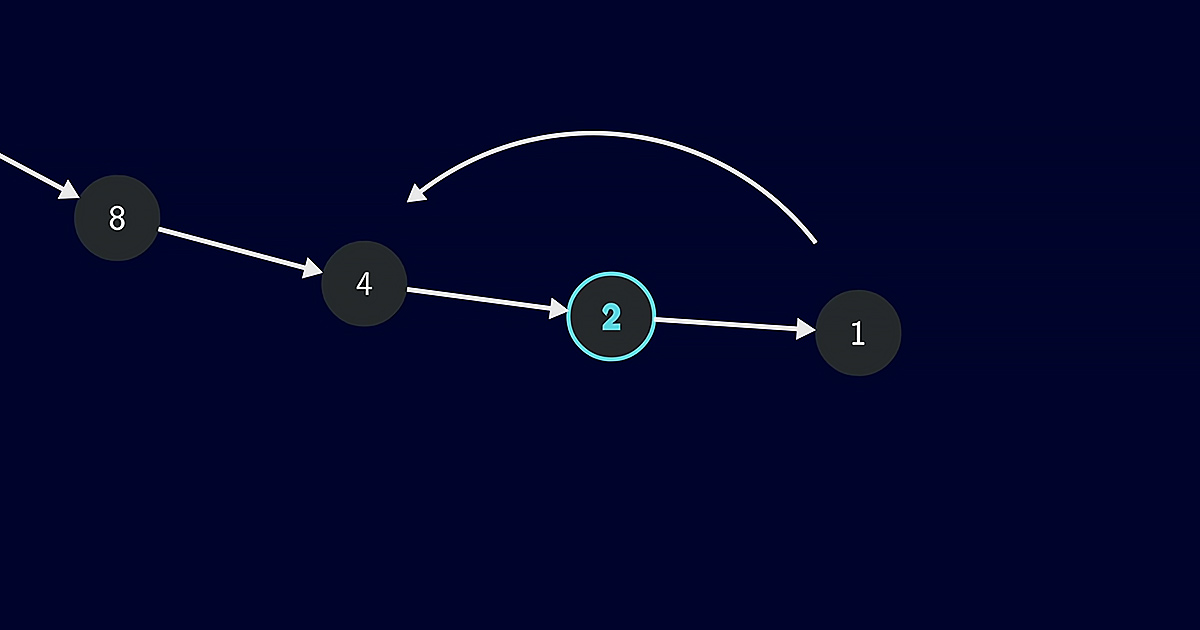# Math problem is easy to understand, but notoriously difficult to solveOne of the most difficult problems in mathematics is known as the Collatz Conjecture or 3x + 1. You take any positive integer and apply specific rules according to whether the number is odd or even.Eventually, you get stuck in a loop of 4, 2, 1, then back to 4. The graphical representation shows that although the numbers display a hailstone pattern, the overall trend is always downward.

This follows a common trend found in given datasets from the stock market to Fibonacci numbers called Benford’s Law. This principle is applied to confirm the absence of tax fraud and voter fraud in elections.Mathematicians warn newbies to steer clear of the Collatz Conjecture as it is a black hole that will steal your life and drive you insane. So far, nearly three quintillion numbers have been tested, with all of them leading to the same loop.

Mathematicians assume that if there is a number that breaks the pattern, it most likely will comprise over 186 billion digits. This number has not yet been discovered by mankind.The Collatz Conjecture cannot be proven true using direct methods, but a counterexample may exist. This is another way of looking at the puzzling math problem without directly proving it true.

The world of mathematics is a fascinating place. It is mind-blowing that a simple little equation such as 3x + 1 is the bane of mathematicians’ existence all over the world.

###### If you liked this, share it with a friend.